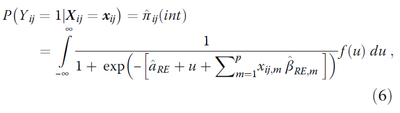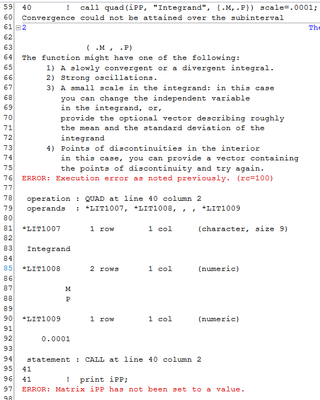## Integrating over a non-normal distribution

I am trying to program correct marginal Predicted Probabilities (PP) from a multi-level logistic regression output.  Pavlou (2015)  has a formula attributed to Skrondal  (2009) where row-level PPs need to be integrated over the distribution of random effects:Expression in the square brackets -- except for 'u' -- is marginal linear predictor.  Random effect (RI) gets added to that expression and the whole thing gets translated into PP.  That entity, then, is integrated over the distribution of random effects with the mean of zero and standard deviation being the variance of the random effecs from the model inquestion.

I am a novice IML programmer and, for the life of me, can't figure out how to program this in SAS.  My variances of RIs range from 0.1 to 0.5 and I don't  even know where to put that in the QUAD() funcion.  The best shot is below.  Any advice is appreciated.

Would averaging PP over all sample RIs be close enough to the integration approach?  I am working with anywhere from 150 to over 1,000 random units.

```proc IML;
start IntRI(RI);
PP = 0.1;
lgt = log(PP/(1-PP));
return( (1 + exp(-(lgt+RI)))**-1 );
finish;

print iPP;
quit;
```
1 ACCEPTED SOLUTION

Accepted Solutions

## Re: Integrating over a non-normal distribution

I don't understand the formula in terms of the random effects model. It looks like it is for a single random effect, but you keep referring to random effects (plural).

However, I can offer assistance with evaluating the integral. Everything except the u and f(u) is constant, so specify the PDF of u in the integrand. For example, if f is the normal density function with mean 0 and the standard deviation of the random effect is sigma=0.73, then you would use the following code to evaluate the integral at a specific point in the space of the explanatory variables:

``````proc IML;
beta = {2.4, -2, 3};  /* coeffs of logistic regression (Intercept, beta_x, beta_y)*/
X =    {1  0.5 0.7};  /* evaluate model at (x,y)=(0.5 0.7) [add intercept to design] */
eta = X*beta;         /* linear predictor at (x,y) */

start Integrand(u) global(eta);
L = logistic(u + eta);
fu = pdf("Normal", u, 0, 0.73);  /* specify f(u) here. eg, PDF for N(0,0.73) */
return( L * fu );
finish;

print iPP;``````

11 REPLIES 11

## Re: Integrating over a non-normal distribution

I don't understand the formula in terms of the random effects model. It looks like it is for a single random effect, but you keep referring to random effects (plural).

However, I can offer assistance with evaluating the integral. Everything except the u and f(u) is constant, so specify the PDF of u in the integrand. For example, if f is the normal density function with mean 0 and the standard deviation of the random effect is sigma=0.73, then you would use the following code to evaluate the integral at a specific point in the space of the explanatory variables:

``````proc IML;
beta = {2.4, -2, 3};  /* coeffs of logistic regression (Intercept, beta_x, beta_y)*/
X =    {1  0.5 0.7};  /* evaluate model at (x,y)=(0.5 0.7) [add intercept to design] */
eta = X*beta;         /* linear predictor at (x,y) */

start Integrand(u) global(eta);
L = logistic(u + eta);
fu = pdf("Normal", u, 0, 0.73);  /* specify f(u) here. eg, PDF for N(0,0.73) */
return( L * fu );
finish;

print iPP;``````

## Re: Integrating over a non-normal distribution

Thanks so much, Rick.

Extra background detail.  There is a problem with marginal observation-level Predicted Probabilities from logistic regression when random effects are involved.  Straight up linear predictor expressed as probability is systematically different from the 'correct' value.  See this for more detail (https://bmcmedresmethodol.biomedcentral.com/articles/10.1186/s12874-015-0046-6).

The correct way to calculated marginal probabilities according to the reference and the formula I pasted, is to integrate Predicted Probability for each row over the distribution of all random intervals in the model.  I wish SAS had this correction built in into all PROCs that do multi-level regression.  I requested that feature.  In the absence of it, my starting point is a marginal NOBLUP Predicted Probability value for each row in the model from GLIMMIX, for example.  From there, I back-transform it to linear predictor so that the LP could be integrated over the distribution of random effects or Random Intervals (the u term).  For each row in the model (patients), the Predicted Probability needs to be corrected using the distribution of random intercepts (hospitals).

Normal integral QUAD() gives me AUC for the transformation without any density weights.  What you shared seems to do the trick.  I tested a couple of values and it seems to correctly increase the input for values below 0.5 and decreases them for values above .5.  I modified the code slightly and now just need to figure out how to apply it to an entire column of Predicted Probability values.  I think I can simplify this a little bit by outputing Linear Predictors from the model rather than Predicted Probabilities -- skip the `iLink` option.

```proc IML;
PredProb = .73;              /* Marginal Predicted Probability from RE GLMM */
Eta 	 = log(PP / (1-PP)); /* Eta = linear predictor                      */

start Integrand(u) global(eta);
L  = logistic(u + eta);
fu = pdf("Normal", u, 0, 0.2);  /* specify f(u) here. eg, PDF for N(0,0.20) */
return( L * fu );
finish;

print PP Eta iPP;
quit;
```

Thanks again.  Happy to discuss further.  Hope you can put in a good word with SAS development teams to add this correction to the SAS/STAT core PROCS.   Would love to hear if you disagree with the diagnosis for marginal PPs.

## Re: Integrating over a non-normal distribution

Oh, and one more thing, I think the notation in the formula is what's throwing you off.  "RE" subscripts for alpha and beta signify that it's a Random Effects model rather than that alpha and beta are random effects coefficients.  Alpha and Beta are fixed.  U is the random intercept.

## Re: Integrating over a non-normal distribution

And I am a total IML idiot.  Why is this code not working?

```proc iml;
use SAShelp.class;

PP	= Age/100;
Eta	= log(PP / (1-PP));

start Integrand(u) global(eta);
L  = logistic(u + eta);
fu = pdf("Normal", u, 0, 0.2);
return( L * fu );
finish;

print iPP;

```## Re: Integrating over a non-normal distribution

It's okay to have questions. Especially when the software or technique is new to you. We were all beginners once.

In your example, Age is a vector. Therefore, ETA is a vector. The integrand requires a scalar value. If you want to evaluate the integral for each student's age, you can do the following:

``````
proc iml;
use SAShelp.class;

PP	= Age/100;
EtaVec	= log(PP / (1-PP));

start Integrand(u) global(eta);
L  = logistic(u + eta);
fu = pdf("Normal", u, 0, 0.2);
return( L # fu );
finish;

Integral = j(nrow(EtaVec), 1);
do i = 1 to nrow(EtaVec);
Eta = EtaVec[i,];   /* get i_th row */
Integral[i] = iPP;
end;
print Age EtaVec Integral;

``````

## Re: Integrating over a non-normal distribution

You are too kind.  Much obliged!  Thanks!

It's a challenge to transition from decades of just columns, rows and cells to vectors and arrays.

## Re: Integrating over a non-normal distribution

Yes, it certainly is. It can be frustrating. It takes time, patience, and hard work. But hopefully we can help help by answering your questions.

If you decide that you want to learn more about programming in the SAS IML language, here are some helpful general tips: Tips for learning the SAS/IML language - The DO Loop

## Re: Integrating over a non-normal distribution

Thanks.  Even better: I bought YOUR book 🙂

## Re: Integrating over a non-normal distribution

Hello, I am interested in this field. But the URL you pasted seems not working and does not link to any material. Could you paste the URL again or report the details (title, author, etc.) of the paper? Thank you!

## Re: Integrating over a non-normal distribution

Closing parenthesis got included in the link. Remove it and the link works. Just checked.

<>
[12874.jpg]
A note on obtaining correct marginal predictions from a random intercepts model for binary outcomes - BMC Medical Research Methodology<>
bmcmedresmethodol.biomedcentral.com<>

## Re: Integrating over a non-normal distribution

Thank you very much!
From The DO Loop